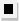Chapter 13.6, Problem 43E### Algebra and Trigonometry (MindTap ...

4th Edition
James Stewart + 2 others
ISBN: 9781305071742

#### Solutions

Chapter
Section### Algebra and Trigonometry (MindTap ...

4th Edition
James Stewart + 2 others
ISBN: 9781305071742
Textbook Problem

# 4 3 - 4 6Factoring Factor using the Binomial Theorem. x 4 + 4 x 3 y + 6 x 2 y 2 + 4 x y 3 + y 4

To determine

To find:

Factor x4+4x3y+6x2y2+4xy3+y4 using Binomial theorem

Explanation

Given:

The expression x4+4x3y+6x2y2+4xy3+y4

Approach:

Deduce the exponent n of the binomial from number of terms in the given expression. And then compare the given expression to (a+b)n and solve for a,b.

Calculation:

The given expression has 5 terms and we know a binomial with exponent n has n+1 terms in its binomial expansion. Hence the binomial exponent corresponding to given expression is n=4

(a+b)4=a4+(41)a

### Still sussing out bartleby?

Check out a sample textbook solution.

See a sample solution

#### The Solution to Your Study Problems

Bartleby provides explanations to thousands of textbook problems written by our experts, many with advanced degrees!

Get Started